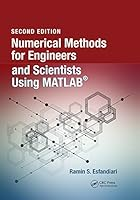# Numerical Methods for Engineers and Scientists Using MATLAB, 2nd Edition0 Reviews
2017-04-25
493 pages

## Book Description

This book provides a pragmatic, methodical and easy-to-follow presentation of numerical methods and their effective implementation using , which is introduced at the outset. The author introduces techniques for solving equations of a single variable and of equations, followed by curve fitting and interpolation of data. The book also provides detailed coverage of numerical differentiation and , as well as numerical solutions of initial-value and boundary-value problems. The author then presents the numerical solution of the eigenvalue problem, which entails approximation of a few or all eigenvalues of a . The last chapter is devoted to numerical solutions of partial differential equations that arise in and science. Each method is accompanied by at least one fully worked-out example showing essential details involved in preliminary hand calculations, as well as computations in MATLAB. This thoroughly-researched resource:

Chapter 1. Background And Introduction
Chapter 2. Introduction To Matlab®
Chapter 3. Numerical Solution Of Equations Of A Single Variable
Chapter 4. Numerical Solution Of Systems Of Equations
Chapter 5. Curve Fitting And Interpolation
Chapter 6. Numerical Differentiation And Integration
Chapter 7. Numerical Solution Of Initial-Value Problems
Chapter 8. Numerical Solution Of Boundary-Value Problems
Chapter 9. Matrix Eigenvalue Problem
Chapter 10. Numerical Solution Of Partial Differential Equations

## Book Details

• Title: Numerical Methods for Engineers and Scientists Using MATLAB, 2nd Edition
• Author:
• Length: 493 pages
• Edition: 2
• Language: English
• Publisher:
• Publication Date: 2017-04-25
• ISBN-10: B07114YM1S